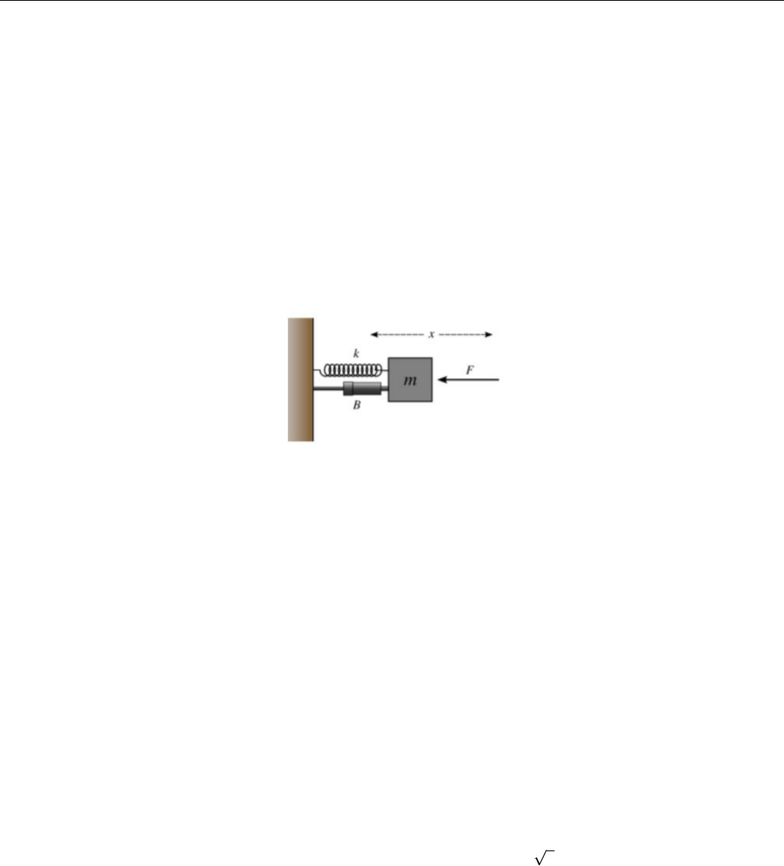Study Guides (380,000)
US (220,000)
CU-Boulder (1,000)
APPM (500)
APPM 2360 (100)
All (100)
Midterm

# appm2360spring2016exam3Exam

Department
Applied Math
Course Code
APPM 2360
Professor
All
Study Guide
Midterm

This preview shows half of the first page. to view the full 3 pages of the document.APPM 2360 Exam 3 Spring 2016
ON THE FRONT OF YOUR BLUEBOOK write: (1) your name, (2) your student ID number, (3) recitation section, (4) your
instructor’s name, and (5) a grading table. Books, class notes, cell phones, and calculators are NOT permitted. A one-page
one-sided crib sheet is allowed.
1. (30 points) For the following two ODE’s, (i) ﬁnd the general solution (ignoring initial conditions) and (ii) the unique
solution satisfying the initial conditions provided.
(a) (15 points)
y′′
5y
6y= 0, y(0) = 2, y(0) = 5
(b) (15 points)
y′′ + 2y+y= 0, y(0) = 0, y(0) = 1
2 (30 points) Consider the harmonic oscillator system:
Assume that the mass of the block is m= 2, the spring constant k= 4, and that the equilibrium position of the block is x= 0.
Both the damping constant band the external force F(t)can vary. Let x(t)denote the position of the block at time t. The initial
conditions are x(0) = 1 and x(0) = 1.
(a) (2 points) Write down the differential equation for x(t), governing the motion of the block, using the supplied values of
mand k.
(b) (8 points) Suppose the external force is zero and let b= 4. Write down the differential equation and ﬁnd the solution
x(t)using the supplied initial conditions. Describe the motion of the block.
(c) (14 points) Suppose no damping is present (b= 0). An external force F(t) = cos(t)is applied to the block. Write down
the differential equation and derive the solution x(t)using the supplied initial conditions. Describe the motion of the
block.
(d) (6 points) Solving for x(t)is not necessary for this question.
(I) (3 points) Suppose that F= 0 and we would like to design the damper so the block returns as fast as possible to the
equilibrium position and ceases oscillation. What must the damping constant be (or what range of values can it take) for
this to be the case?
(II) (3 points) Suppose no damping is present (b= 0) and a force F(t) = cos 2tis applied. Describe the motion of
the block in this case.
CONTINUED
###### You're Reading a Preview

Unlock to view full version